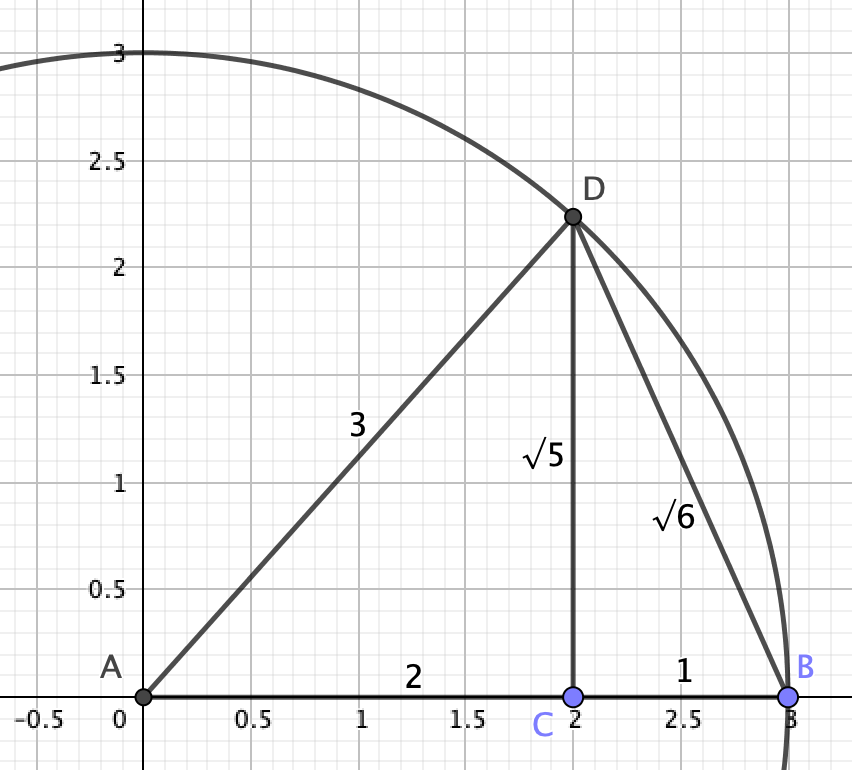On Twitter, @RuedigerSimpson pointed me at an episode of My Favourite Theorem in which @FawnPNguyen mentioned a method for constructing $\sqrt{7}$:

• draw a circle of radius 4
• construct a perpendicular to the radius at a distance of 3 from the centre
• the distance between the base of the perpendicular and where it meets the circle is $\sqrt{7}$, because $4^2 - 3^2 = 7$.

Very nice! The square root of any odd number can be constructed a similar way - $\sqrt{2n+1} = \sqrt{(n+1)^2 - n^2}$, by the difference of two squares.

Similarly, multiples of 4 are easy pickings: $\sqrt{4n} = (n+1)^2 - (n-1)^2$

Which leaves only numbers of the form $4n+2$ - which can’t be expressed immediately as the difference of two squares. But can such numbers be constructed similarly?

## Bisection!

A simple way to construct, say $\sqrt{6}$ would be to construct $\sqrt{24}$ using the method above (a circle of radius 7 and a perpendicular 5 units away) and bisect the perpendicular. Since $\sqrt{6} = \frac{1}{2}\sqrt{24}$, we’ve got our distance!

But Simon didn’t like that. Can it be done without bisection?

## No bisection!

I’m quite pleased with my alternative method, which I’m told is based on the Spiral of Theodorus, although it’s rather less involved.

If you construct $\sqrt{5}$ using the method above, with a circle of 3 units and a perpendicular of 2, there’s a second right-angled triangle you can use: the one to the right of the perpendicular! The perpendicular has length $\sqrt{5}$, the base has length 1, so the hypotenuse has length $\sqrt{6}$!I thought that was a neat bit of geometry. I don’t for a second imagine it’s new (it’s probably in Euclid if I could be bothered to look), but it was pretty enough a solution to make me grin.

Do you know of any other nice methods?